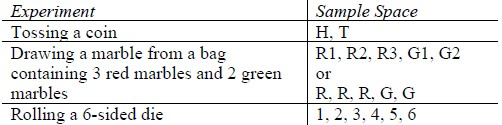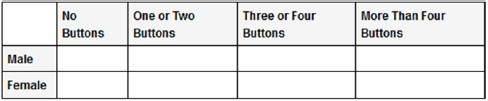# MA.7.DP.2.3Export Print
Find the theoretical probability of an event related to a simple experiment.

### Clarifications

Clarification 1: Instruction includes representing probability as a fraction, percentage or decimal.

Clarification 2: Simple experiments include tossing a fair coin, rolling a fair die, picking a card randomly from a deck, picking marbles randomly from a bag and spinning a fair spinner.

General Information
Subject Area: Mathematics (B.E.S.T.)
Strand: Data Analysis and Probability
Status: State Board Approved

## Benchmark Instructional Guide

### Terms from the K-12 Glossary

• Event
• Theoretical Probability

### Vertical Alignment

Previous Benchmarks

Next Benchmarks

### Purpose and Instructional Strategies

In grade 7, students find the theoretical probability of an event related to a simple experiment, and in grade 8, they will find the theoretical probability of an event related to a repeated experiment.
• Instruction builds on finding sample spaces from MA.7.DP.2.1. Have students discuss their understanding of the words “theoretical” and “probability” to build toward a formal definition of theoretical probability.
• When finding theoretical probability, have students work from their sample space. Doing so will lead to the understanding that since experiments for this benchmark are fair, the probability of an event is equivalent to $\frac{\text{number of outcomes in the event}}{\text{number of outcomes in the sample space}}$.
• For example, if rolling a fair 6-sided die, the sample space is {1, 2, 3, 4, 5, 6,}. If one wants to find $P$(), students can circle all of the odd numbers from the sample space to determine the probability as , or 0.5.
• While the benchmark does focus on fair experiments, instruction could include spinners with unequal sections making the connection to angle measures and to circle graphs.
• Instruction focuses on the simple experiments listed in Clarification 2.
• For example, when tossing a coin with one side colored yellow and the other side colored red, $P$() = 0.
• For example, when rolling a 10-sided die, $P$( 3) = 0.7.
• For example, when picking a card from a deck that contains each of the letters of the alphabet, $P$() = $\frac{\text{21}}{\text{26}}$.
• For example, when picking a tiles from a bag that contains a set of chess pieces, $P$() = 50%.
• For example, when spinning a spinner that contains 5 sections where two of the sections are green and the remaining sections are red, white and blue,
$P$() = $\frac{\text{3}}{\text{5}}$.

### Common Misconceptions or Errors

• Students may incorrectly convert forms of probability between fractions and percentages. To address this misconception, scaffold with more familiar values initially to facilitate the interpretation.
• Students may incorrectly count outcomes when one outcome appears more than once in the sample space.
• For example, if the sample space is {red, red, blue} and one wants to find $P$($red$), a student may incorrectly state $\frac{\text{1}}{\text{2}}$ or $\frac{\text{1}}{\text{3}}$ instead of $\frac{\text{2}}{\text{3}}$.

### Strategies to Support Tiered Instruction

• Teacher provides instruction in converting between fractions and percentages, by using more familiar values.
• For example, $\frac{\text{1}}{\text{2}}$ = 50%, $\frac{\text{1}}{\text{4}}$ = 25%, $\frac{\text{1}}{\text{3}}$ ≈ 33.3%, etc.
• Teacher co-creates a T-chart (like the one below) to list the experiment and the sample space necessary for the examples provided.Look at the shirt you are wearing today and determine how many buttons it has. Then complete the following table for all the members of your class.Suppose each student writes his or her name on an index card, and one card is selected randomly.
• Part A. What is the probability that the student whose card is selected is wearing a shirt with no buttons?
• Part B. What is the probability that the student whose card is selected is female and is wearing a shirt with two or fewer buttons?

There is only one question on the next quiz and it will be true or false.
• Part A. If a student randomly answers the question, what is the probability of earning a score of 100%?
• Part B. What is the probability of earning a 50%?

### Instructional Items

Instructional Item 1
What is the probability of choosing a 9 from a standard deck of 52 cards?

Instructional Item 2
There are 7 red, 5 blue and 12 green marbles in a bag.
• Part A. What is the probability of choosing a red marble?
• Part B. What is the probability of not choosing a green marble?
• Part C. What is the probability of choosing a yellow marble?
• Part D. What is the probability of choosing a red or blue marble?

*The strategies, tasks and items included in the B1G-M are examples and should not be considered comprehensive.

## Related Courses

This benchmark is part of these courses.
1205020: M/J Accelerated Mathematics Grade 6 (Specifically in versions: 2014 - 2015, 2015 - 2020, 2020 - 2022, 2022 and beyond (current))
1205040: M/J Grade 7 Mathematics (Specifically in versions: 2014 - 2015, 2015 - 2022, 2022 and beyond (current))
1204000: M/J Foundational Skills in Mathematics 6-8 (Specifically in versions: 2014 - 2015, 2015 - 2022, 2022 and beyond (current))
7812020: Access M/J Grade 7 Mathematics (Specifically in versions: 2014 - 2015, 2015 - 2018, 2018 - 2019, 2019 - 2022, 2022 and beyond (current))

## Related Access Points

Alternate version of this benchmark for students with significant cognitive disabilities.
MA.7.DP.2.AP.3: Determine the theoretical probability of a simple chance event.

## Related Resources

Vetted resources educators can use to teach the concepts and skills in this benchmark.

## Formative Assessments

Game of Chance:

Students are asked to estimate the frequency of an event given its probability and explain why an expected frequency might differ from an observed frequency.

Type: Formative Assessment

Technical Difficulties:

Students are given a scenario and asked to determine the probability of two different events.

Type: Formative Assessment

## Lesson Plans

Genetics Has Gone to the Dogs!:

This lesson uses pooches to teach about pedigrees and the impact of artificial selection on individuals and populations as well as to drive home math concepts already discussed in lessons on Punnet squares.

Type: Lesson Plan

Independent Compound Probability:

During this lesson, students will use Punnett Squares to determine the probability of an offspring's characteristics.

Type: Lesson Plan

Probabilities and Punnett Squares:

Students simulate the process of meiosis for an alien society. The students choose physical characteristics for hair, nose and eyes corresponding to genes and then generate two alien babies. Then pictures of the parents and babies are drawn, with similarities and differences noted and explained.

Type: Lesson Plan

## Perspectives Video: Expert

History of Probability and the Problem of Points:

What was the first question that started probability theory?

Type: Perspectives Video: Expert

## Text Resource

Shuffling Shenanigans:

This informational text resource is intended to support reading in the content area. A student in love with magic card tricks asks and answers his own math questions after pursuing a career as a mathematician in order to solve them. How many times must a deck be shuffled to achieve a truly random mix of cards? The answer lies within.

Type: Text Resource

## Tutorials

Finding Probability:

This video demonstrates several examples of finding probability of random events.

Type: Tutorial

Finding Probability of a Simple Event:

This video demonstrates how to find the probability of a simple event.

Type: Tutorial

## MFAS Formative Assessments

Game of Chance:

Students are asked to estimate the frequency of an event given its probability and explain why an expected frequency might differ from an observed frequency.

Technical Difficulties:

Students are given a scenario and asked to determine the probability of two different events.

## Student Resources

Vetted resources students can use to learn the concepts and skills in this benchmark.

## Tutorials

Finding Probability:

This video demonstrates several examples of finding probability of random events.

Type: Tutorial

Finding Probability of a Simple Event:

This video demonstrates how to find the probability of a simple event.

Type: Tutorial

## Parent Resources

Vetted resources caregivers can use to help students learn the concepts and skills in this benchmark.